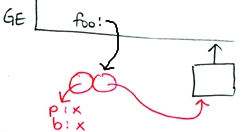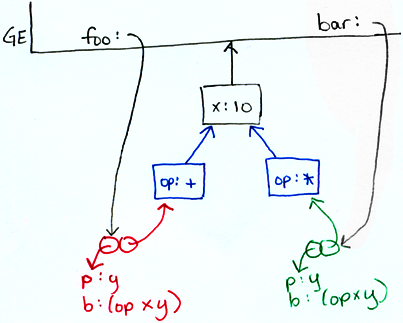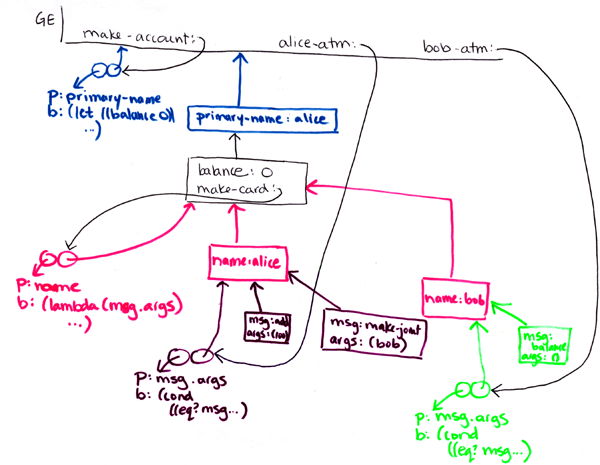```recitation 16: message passing

========================================================================
Warmup exercises:

* write a procedure max that takes in 2 or more numbers and
returns the largest number.  (Hint: you'll need to use ". args")

(max 3 1 6 4 2) -> 6
(max 3 1) -> 3

(define (max a b . args)
(fold-right (lambda (x m) (if (> x m) x m))
a
(cons b args)))

* write a procedure add-numbers-in-lists that takes in two lists of
numbers and adds them all together.  Use apply.

(add-numbers-in-lists (list 1 2 3) (list 4 5 6)) -> 21

(apply + (append a b)))

* generalize add-numbers-in-lists to take 1 or more lists of numbers.

(add-numbers-in-lists (list 1 2 3) (list 4) (list) (list 5 6)) -> 21

(apply + (fold-right append a lists)))

* write a procedure multi-list-map that takes in an op on n arguments
and n lists (where n >= 1) and does the right thing.  (Hint: you'll
need to use ". args" and apply)

(multi-list-map max
(list 0 2 4 6 8)
(list 8 6 4 2 0)
(list 5 5 5 5 5)) -> (8 6 5 6 8)

(define (multi-list-map op lst . lists)
(if (null? lst)
nil
(cons (apply op (map car (cons lst lists)))
(apply multi-list-map
(cons op (map cdr (cons lst lists)))))))

========================================================================
how do we build a system to apply generic operations on different types of data?

* tagged data & "dispatch on type"

* message passing
- make the data into "smart" procedures with state
- procedures can "store" data (environment pointer part)
- methods for manipulating data

* object oriented programming
- message passing + lots of goodies
- more on Thursday & Friday
- next project!

how easy is it to break the system into modules?
how easy is it to extend the system?
organize the types & operations into a table, then
group by row or by column, either by operation or data type
class vs. instance

========================================================================
Write code that results in this environment diagram (some things may not be drawn):
``````
(define foo (let () (lambda (x) x)))
- OR -
(define foo ((lambda () (lambda (x) x))))

========================================================================
Write code that results in this environment diagram (some things may not be drawn):
``````
(define thing (let ((x 10)) (lambda (op) (lambda (y) (op x y)))))
(define foo (thing +))
(define bar (thing *))

========================================================================
Let's write a bank atm system in message passing style with the following behavior:

(define alice-atm (make-account 'alice))

(alice-atm 'balance) => 0
(alice-atm 'balance) => 100
(alice-atm 'subtract 20)
(alice-atm 'balance) => 80

(alice-atm 'name) => alice
(alice-atm 'primary-name) => alice

(define bob-atm (alice-atm 'make-joint 'bob))
(bob-atm 'name) => bob
(bob-atm 'primary-name) => alice

(bob-atm 'balance) => 80
(bob-atm 'balance) => 180
(bob-atm 'subtract 20)
(bob-atm 'balance) => 160

(alice-atm 'balance) => 160

first sketch the environment diagram you'll need to implement, then write the code.

(define (make-account primary-name)
(let ((balance 0))
(define (make-card name)
(lambda (msg . args)
(cond ((eq? msg 'name) name)
((eq? msg 'primary-name) primary-name)
((eq? msg 'balance) balance)
((eq? msg 'add) (set! balance (+ balance (car args))))
((eq? msg 'subtract) (set! balance (- balance (car args))))
((eq? msg 'make-joint) (make-card (car args)))
(else "unknown message"))))
(make-card primary-name)))

The environment diagram for the above solution:
``````========================================================================
```